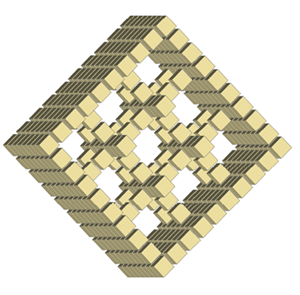# The Eureka Moment – 3/7

### The Eureka moment of further discovery.

Seven years later in 1985, when looking through some of my ideas, I came across my unresolved number cube and had another look, this time considering another math, true to the math of the original square but not based on multiplying numbers. I suddenly found the secret and actually shouted Eureka out loud, as the whole inner number arrangement fell into place, within a matter of minutes. As I discovered throughout my life, lateral thinking moments are instantaneous, and the time taken afterward, even minutes as above, is the time required to check this instantaneous information spike, against the whole puzzle.

I used the second true logic of the ‘Arabic’ number square i.e adding the outer numbers on the X and Y axes with the inner numbers along each column to reveal the numbers within each inner layer (or level) of the cube. However to obtain the inner numbers, the only starting point, having only the outer numbers known for each layer, was the addition of the second numbers along both axes. For example, as shown in the diagramme for the Second Layer (or level) below, the outer numbers are 2, 4, 6, 8, 1, 3, 5, 7 from each corner of the X and Y axes. To start, the way I found to calculate the inner numbers, was to add the second outer numbers from each corner i.e. 4 + 4 = 8 and 5 + 5 = 10 and 1 + 0 = 1. Once the two of these four inner corner numbers (i.e. 8 and 1) are established, the remaining inner numbers can revealed by adding the outer number to this number to obtain the next number in the column (eg 4 + 8 = 12 = 1 + 2 = 3), and then to add the same outer number with this new number to reveal the next ( eg 4 + 3 = 7) and so forth along both axes, resulting in all numbers being revealed for each layer of the cube. I also noticed at this point that the outer numbers doubled become the next numbers in.

I find it to be a most fortunate coincidence that the first inner corner numbers (for example numbers 1 and 8 in the Second layer) are achievable from two different calculations. For example the number 1 is achieved by adding the second number in from the bottom left corner i.e. 5 + 5 = 10 = 1 + 0 = 1 but is also achieved by adding the outer 4, at the top of the same column, with the preceding number 6 i.e. 4 + 6 = 10 = 1 + 0 = 1. The adding logic of the initial square follows through all inner layers (levels) of the cube.

The layout pattern for each number changes for each layer, but the number couplings remain true and constant through the cube i.e. 1 with 8, 2 with 7, 3 with 6 and 4 with 5. Note that these numbers work right through the cube, identically from all six faces of the cube. The number 9 forms a complete outer shell of 9’s around the cube.

The following images are shown as the levels of each layer of the cube in slices, starting with the amended ‘Arabic’ magic square as level one (note that level zero and level nine, shown last, are the outer shell faces of the Ant Cube and consist of all nines) through to level nine, which is ten layers or levels in all. Whichever way you turn the cube, these levels apply equally from top to bottom, bottom to top and any side to opposite side. The 1000 number cube is multi directional without any numbers clashing. Remember that these numbers have not been made up, but are inherent in the mathematics that I deduced from the original ‘Arabic’ Magic Square, into three dimensions.

In the image layouts below, Level one is the multi-directional square based on the ‘Arabic’ Magic Square but with the full perimeter of 9’s that I deduced from this number square. I have used colours to highlight the number patterns of each number. The coupling numbers are in contrasting colour for ease of identification.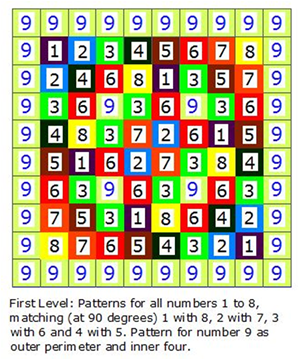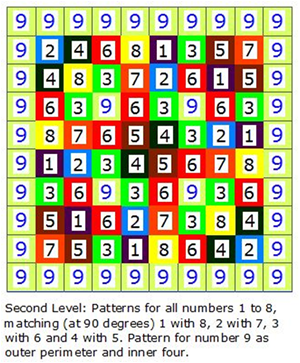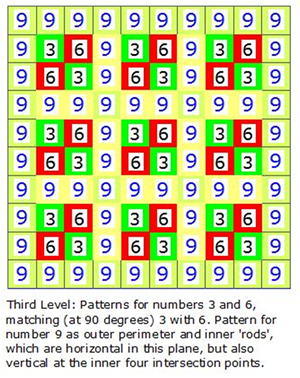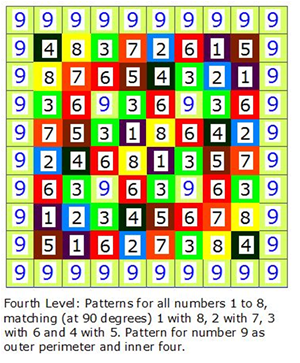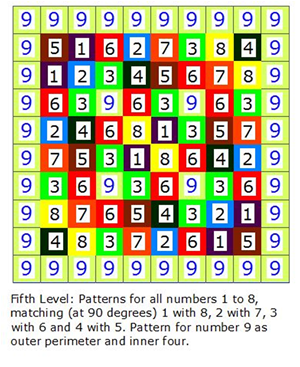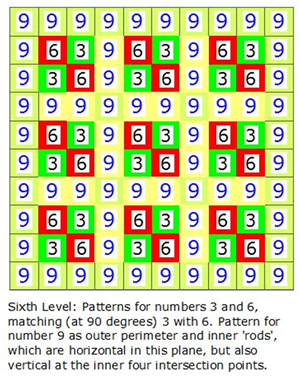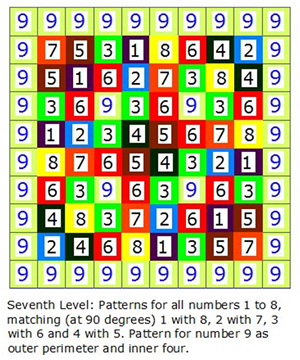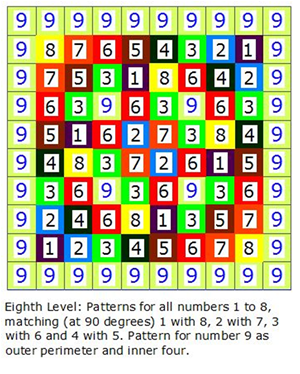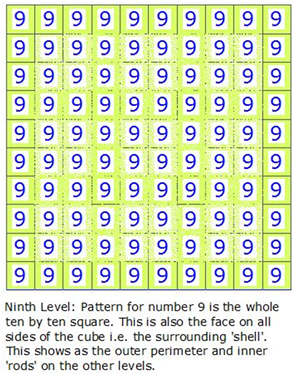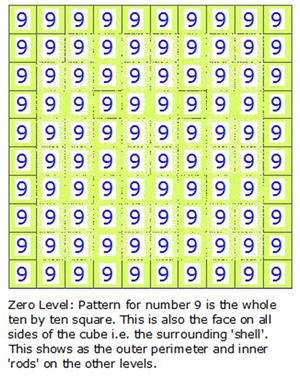Fig 5. The Zero and Ninth levels are shown together for better visual clarity. Each of the six outer faces of the cube looks like this, as an outer layer of 9’s.

The Structural Quality of Number 9: The number nine, apart from forming a complete outer shell around the cube, reveals another major difference from the pattern layouts of the other numbers; the inner four nines shown in the original 2D magic square, repeated on each of the six faces of the cube, run through the 3D cube in straight rods, from face to face, each rod of 9’s intersecting at two points within the cube and connecting with the outer shell. These twelve rods (note that the two opposite faces of the cube each share the same four rods of the number 9, so effectively there is a structure of three lots of four rods = twelve rods and not six lots of four rods = twenty four rods) become the connecting structure around which the other number couplings sit.

Fig 6. Within this 1000 number cube the number 9 is revealed as a ‘physical’ 3D structure i.e. 9, 9, 9.In the image on the right, the zero and ninth levels have been left off for clarity.